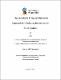## An impedance frequency response approach for analyzing interactions in ac-dc systems2019-08-13
Qi, Yi
##### Abstract
This thesis presents an integrated technique for screening the interaction level between the ac side system and dc side converters. The system resonances in the sub-synchronous and super-synchronous frequency range are identified and stability determined. This approach applies the Generalized Nyquist stability Criterion (GNC) assuming that the frequency dependent impedance responses are available. The frequency responses of the subsystems are obtained using two different techniques. One is an analytical state variable analysis requiring the mathematical model of the system elements; and the other is a simulation-based frequency scanning approach which does not rely on detailed knowledge of the internal system. These two techniques can be applied in different situations. The state variable analysis is useful when a small signal model is derivable, e.g., for the HVdc converter system, and the frequency scanning method is useful if only ‘black boxed’ models are available. For stability analysis, the systems are represented in the dq0 domain which gets rid of the coupling between frequencies. The frequency scanning is conducted on two Electromagnetic Transients (EMT) simulation platforms, the off-line PSCAD/EMTDC and the real-time RTDS. PSCAD/EMTDC uses a highly-accurate bi-valued resistor model for the switches and its scanned impedance response matches the analytically derived result very well. The RTDS uses a more approximate technique that allows high speed simulation. The impedance scans from RTDS also agree with the analytical results showing that the scanning technique is also a mechanism for validating various new models. An important contribution of this research is the development of an improved small signal analytical model of the Line Commutated Converter (LCC). Traditional studies neglected the transient dynamics of the commutation inductor and modeled it as the reactance of fundamental frequency. The model realized here assumes an infinite series-connected 6- pulse converters and regards the 12-pulse converter as one of its sampled versions. With this treatment, the waveforms of the currents and voltages are ripple-free, and the measured angles, i.e., the overlap angle and the extinction angle become continuous. This model is validated by comparing the analytical converter response with the one obtained from the frequency scanning. Moreover, this LCC model is interfaced with an arbitrary external system, where the system stability is determined using GNC. Based on the impedance responses, the ac-dc system is represented as a closed-loop system, in which the dc side impedance is the forward gain and the ac side admittance is the feedback, or vice versa. The GNC is applied on the closed-loop system and a new index “Harmonic Stability Margin” is proposed to indicate how much a gain or other parameter can be scaled so that the system reaches the boundary of instability. As the index can parameterize the ac-dc interaction level, HSM is then used in several case systems to study the Sub-Synchronous Control Interaction (SSCI), the impact of detailed modeling of remote side converter, as well as the multi-infeed system study. All the results are validated by EMT simulations.
##### Keywords
Ac-dc interaction, Impedance response, Electromagnetic Transient (EMT) simulations, Frequency scan, LCC improved model, Generalized Nyquist stability Criterion (GNC), Harmonic Stability Margin (HSM)
##### Citation
Y. Qi, H. Zhao, S. Fan, A. M. Gole, H. Ding and I. T. Fernando, "Small Signal Frequency-Domain Model of a LCC-HVDC Converter Based on an Infinite Series-Converter Approach," in IEEE Transactions on Power Delivery, vol. 34, no. 1, pp. 95-106, Feb. 2019.
Yi Qi, Aniruddha Gole, Hui Ding, Yi Zhang, "Evaluation of the Accuracy of Real-time Digital Simulator Voltage Source Converter Models Determined from Frequency Scanning," in International Conference on Power System Transients (IPST 2017), Seoul, South Korea, June 26-29, 2017.
Yi Qi, Aniruddha Gole, Xiuyu Chen, “Investigation of Subsynchronous Control Interaction for LCC using the Generalized Nyquist Stability Criterion”, The 15th IET 174 international conference on AC and DC Power Transmission, Manchester, Feb 5-7, 2019.